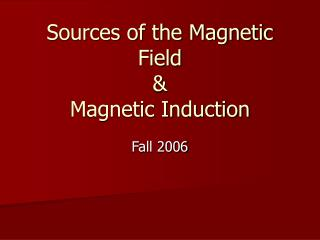Download PresentationSources of the Magnetic Field & Magnetic InductionSources of the Magnetic Field & Magnetic Induction - PowerPoint PPT Presentation

Download PresentationSources of the Magnetic Field & Magnetic Induction
An Image/Link below is provided (as is) to download presentation

Download Policy: Content on the Website is provided to you AS IS for your information and personal use and may not be sold / licensed / shared on other websites without getting consent from its author. While downloading, if for some reason you are not able to download a presentation, the publisher may have deleted the file from their server.

- - - - - - - - - - - - - - - - - - - - - - - - - - - E N D - - - - - - - - - - - - - - - - - - - - - - - - - - -
Presentation Transcript

1. Remember the wire?

2. Try to remember… Coulomb

3. The “Coulomb’s Law” of Magnetism The Law of Biot-Savart A Vector Equation … duck

4. For the Magnetic Field,current “elements” create the field. This is the Law of Biot-Savart This, defines B!

5. Magnetic Field of a Straight Wire • We intimated via magnets that the Magnetic field associated with a straight wire seemed to vary with 1/d. • We can now PROVE this!

6. Using Magnets From the Past

7. Directions: The Right Hand Rule Right-hand rule: Grasp the element in your right hand with your extended thumb pointing in the direction of the current. Your fingers will then naturally curl around in the direction of the magnetic field lines due to that element. Reminder !

8. Let’s Calculate the FIELD Note: For ALL current elements in the wire: ds X r is into the page

9. The Details

10. Moving right along 1/d Verify this.

11. A bit more complicatedA finite wire

12. r q p-q ds P1

13. More P1

14. P2

15. APPLICATION: Find the magnetic field B at point P A Combination of P2 geometries.

16. Center of a Circular Arc of a Wire carrying current

17. ds More arc…

18. The overall field from a circular current loop Sorta looks like a magnet!

19. Iron

20. Howya Do Dat?? No Field at C

21. Force Between Two Current Carrying Straight Parallel Conductors Wire “a” creates a field at wire “b” Current in wire “b” sees a force because it is moving in the magnetic field of “a”.

22. The Calculation

23. Definition of the Ampere The force acting between currents in parallel wires is the basis for the definition of the ampere, which is one of the seven SI base units. The definition, adopted in 1946, is this: The ampere is that constant current which, if maintained in two straight, parallel conductors of infinite length, of negligible circular cross section, and placed 1 m apart in vacuum, would produce on each of these conductors a force of magnitude 2 x 10-7 newton per meter of length. Huh?

24. Ampere’s Law The return of Gauss

25. Remember GAUSS’S LAW?? Surface Integral

26. Gauss’s Law • Made calculations easier than integration over a charge distribution. • Applied to situations of HIGH SYMMETRY. • Gaussian SURFACE had to be defined which was consistent with the geometry. • AMPERE’S Law is the Gauss’ Law of Magnetism! (Sorry)

27. The next few slides have been lifted from Seb Oliveron the internet Whoever he is!

28. Biot-Savart • The “Coulombs Law of Magnetism”

29. Invisible Summary • Biot-Savart Law • (Field produced by wires) • Centre of a wire loop radius R • Centre of a tight Wire Coil with N turns • Distance a from long straight wire • Force between two wires • Definition of Ampere

30. Magnetic Field from a long wire Using Biot-Savart Law r I Take a short vector on a circle, ds B ds Thus the dot product of B & the short vector ds is:

31. Sum B.ds around a circular path r I B Sum this around the whole ring ds Circumference of circle

32. Consider a different path • Field goes as 1/r • Path goes as r. • Integral independent of r i

33. SO, AMPERE’S LAWby SUPERPOSITION: We will do a LINE INTEGRATION Around a closed path or LOOP.

34. Ampere’s Law USE THE RIGHT HAND RULE IN THESE CALCULATIONS

35. The Right Hand Rule .. AGAIN

36. Another Right Hand Rule

37. COMPARE Line Integral Surface Integral

38. Simple Example

39. Field Around a Long Straight Wire

40. Field INSIDE a WireCarrying UNIFORM Current

41. The Calculation Graph ????

42. B R r

43. Procedure • Apply Ampere’s law only to highly symmetrical situations. • Superposition works. • Two wires can be treated separately and the results added (VECTORIALLY!) • The individual parts of the calculation can be handled (usually) without the use of vector calculations because of the symmetry. • THIS IS SORT OF LIKE GAUSS’s LAW WITH AN ATTITUDE!

44. The figure below shows a cross section of an infinite conducting sheet carrying a current per unit x-length of l; the current emerges perpendicularly out of the page. (a) Use the Biot–Savart law and symmetry to show that for all points P above the sheet, and all points P´ below it, the magnetic field B is parallel to the sheet and directed as shown. (b) Use Ampere's law to find B at all points P and P´.

45. FIRST PART Vertical Components Cancel

46. L B Infinite Extent B Apply Ampere to Circuit

47. B Infinite Extent B The “Math” Bds=0 Distance not a factor!

48. A Physical Solenoid

49. Inside the Solenoid For an “INFINITE” (long) solenoid the previous problem and SUPERPOSITION suggests that the field OUTSIDE this solenoid is ZERO!